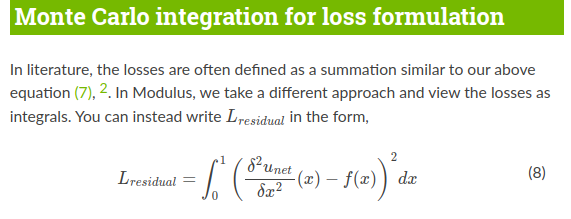# Combining PINN with FVM

Inspired by this paper, I am trying to combine PINN with FVM, but I don’t know how to get the values around the collocation points. For example, \$u_{e}\$ .

Is it possible to get values around collocation points in Modulus other than using Taylor series approximation?
Thanks!

I have not read the paper in full, however something inside of Modulus that could provide some inspiration is our Meshless Finite Derivative nodes which query neighboring stencil points on top of the training points. Derivatives are them computed using finite difference (more information in our user guide).

Seems to be a fairly similar idea to this, but finite difference tends to extend across multiple PDEs more (although FVM is more well suited for flow related problems). Hope this can give you some ideas.

Thanks a lot for your reply, where should I get the source code for `MeshlessFiniteDerivative`?

Inside the Modulus source folder, you can find related code in:

`modulus/eq/derivatives.py`

as well as
`modulus/eq/mfd`

Thanks. And another question, how to use Monte Carlo integration for loss formulation instead of general loss function ? Where I can get the source code for Monte Carlo integration?

I am assuming you are referring to the integral based loss functions (typically used in continuity planes in many of our flow problems). For more information on these please see:

`modulus/domain/constraint/continuous.py` and find the classes `IntegralConstraint` or `IntegralBoundaryConstraint`. In general the idea is each training example is a set of points each with a approximate area they represent, which can then be all summed up for a Monte Carlo integration loss. So a single batch of these integral constraints is actually a set of these point sets.

These use a special loss function which is `IntegralLossNorm` found in `modulus/loss/loss.py`.

Several of examples that are shipped with modulus use these constraints, one I would recommend would be the annular ring problem found in the examples repo under: `annular_ring/annular_ring/annular_ring.py`

Wait, I thought the Monte Carlo integration for loss formulation is the default loss in Modulus.

See this,1 Like

Yes, you’re correct! The default point wise loss is posed in a integral form since it is just the summation of the training points over the domain with a given area size.

@1440590317 if you are not referring to the integral constraint based losses and rather the default loss used in our standard boundary/interior this is enabled by default as @prakhar_sharma mentioned. This is again found in `modulus/loss/loss.py` in class `PointwiseLossNorm`.

1 Like

@ngeneva I used finite volume methods on some equations, which led to some integral terms in the equations. I’m solving an integral-differential equation. My idea is to use Monte Carlo integrals to solve the integral term in the equation, while the differential term is still automatic differentiation. My problem at the moment is that I don’t know how to get the value of the neighborhood around the collocation points.

During the forward execution of all constraints, variables are collected in a variable dictionary: `{x: torch.Tensor, y: torch.Tensor, ...}`. You can probably write a custom constraint or node (like the meshless finite difference node) that can manually build these needed neighborhood collocation points.

Alternatively, if you want to define these collocation points ahead of time on initialization, it sounds like you need a custom dataset. I am not 100% sure of what the exact needs are here. But I would look into extending a dataset or a constraint. The Darcy problems with FNO implement a custom dataset and the super-resolution problem has a custom constraint for reference.

Thanks for your reply. I will try it.

Hi @1440590317,

This is a very interesting use case and we would be interested in chatting about your use case. Would you be interested in discussing with the modulus team to share more details on the use case and we can try to share more insights on how to apply Modulus for you problem. You can reach out to us directly at modulus-team@exchange.nvidia.com

Thanks
Ram